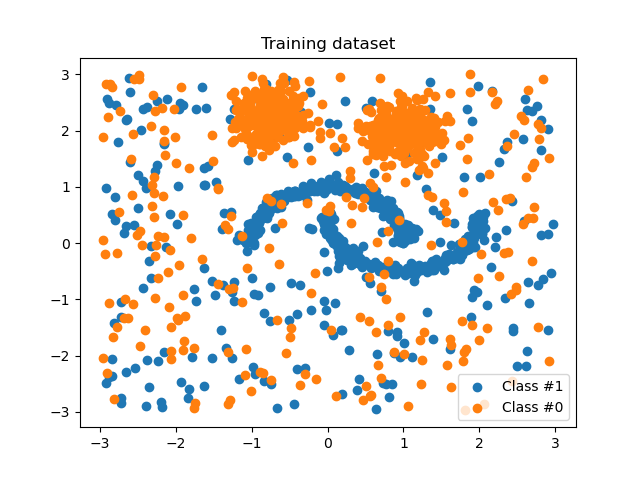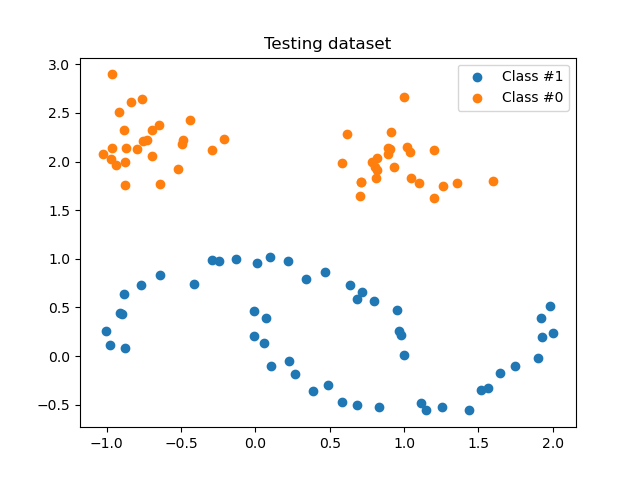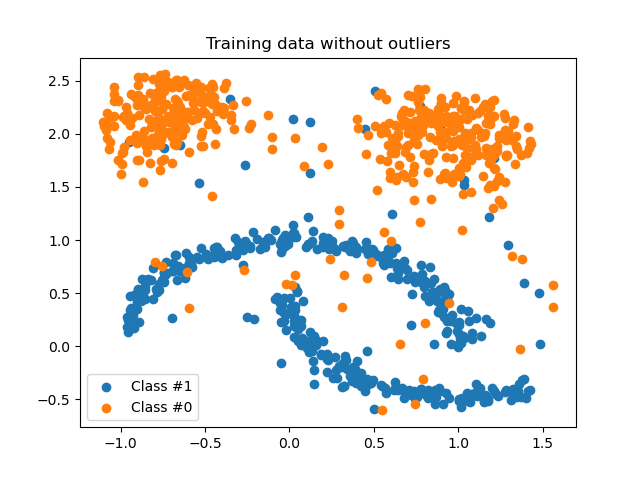# Customized sampler to implement an outlier rejections estimator#

This example illustrates the use of a custom sampler to implement an outlier rejections estimator. It can be used easily within a pipeline in which the number of samples can vary during training, which usually is a limitation of the current scikit-learn pipeline.

```# Authors: Guillaume Lemaitre <g.lemaitre58@gmail.com>

import matplotlib.pyplot as plt
import numpy as np
from sklearn.datasets import make_blobs, make_moons
from sklearn.ensemble import IsolationForest
from sklearn.linear_model import LogisticRegression
from sklearn.metrics import classification_report

from imblearn import FunctionSampler
from imblearn.pipeline import make_pipeline

print(__doc__)

rng = np.random.RandomState(42)

def plot_scatter(X, y, title):
"""Function to plot some data as a scatter plot."""
plt.figure()
plt.scatter(X[y == 1, 0], X[y == 1, 1], label="Class #1")
plt.scatter(X[y == 0, 0], X[y == 0, 1], label="Class #0")
plt.legend()
plt.title(title)
```

## Toy data generation#

We are generating some non Gaussian data set contaminated with some unform noise.

```moons, _ = make_moons(n_samples=500, noise=0.05)
blobs, _ = make_blobs(
n_samples=500, centers=[(-0.75, 2.25), (1.0, 2.0)], cluster_std=0.25
)
outliers = rng.uniform(low=-3, high=3, size=(500, 2))
X_train = np.vstack([moons, blobs, outliers])
y_train = np.hstack(
[
np.ones(moons.shape, dtype=np.int8),
np.zeros(blobs.shape, dtype=np.int8),
rng.randint(0, 2, size=outliers.shape, dtype=np.int8),
]
)

plot_scatter(X_train, y_train, "Training dataset")
```We will generate some cleaned test data without outliers.

```moons, _ = make_moons(n_samples=50, noise=0.05)
blobs, _ = make_blobs(
n_samples=50, centers=[(-0.75, 2.25), (1.0, 2.0)], cluster_std=0.25
)
X_test = np.vstack([moons, blobs])
y_test = np.hstack(
[np.ones(moons.shape, dtype=np.int8), np.zeros(blobs.shape, dtype=np.int8)]
)

plot_scatter(X_test, y_test, "Testing dataset")
```## How to use the `FunctionSampler`#

We first define a function which will use `IsolationForest` to eliminate some outliers from our dataset during training. The function passed to the `FunctionSampler` will be called when using the method `fit_resample`.

```def outlier_rejection(X, y):
"""This will be our function used to resample our dataset."""
model = IsolationForest(max_samples=100, contamination=0.4, random_state=rng)
model.fit(X)
y_pred = model.predict(X)
return X[y_pred == 1], y[y_pred == 1]

reject_sampler = FunctionSampler(func=outlier_rejection)
X_inliers, y_inliers = reject_sampler.fit_resample(X_train, y_train)
plot_scatter(X_inliers, y_inliers, "Training data without outliers")
```## Integrate it within a pipeline#

By elimnating outliers before the training, the classifier will be less affected during the prediction.

```pipe = make_pipeline(
FunctionSampler(func=outlier_rejection),
LogisticRegression(solver="lbfgs", multi_class="auto", random_state=rng),
)
y_pred = pipe.fit(X_train, y_train).predict(X_test)
print(classification_report(y_test, y_pred))

clf = LogisticRegression(solver="lbfgs", multi_class="auto", random_state=rng)
y_pred = clf.fit(X_train, y_train).predict(X_test)
print(classification_report(y_test, y_pred))

plt.show()
```
```              precision    recall  f1-score   support

0       1.00      1.00      1.00        50
1       1.00      1.00      1.00        50

accuracy                           1.00       100
macro avg       1.00      1.00      1.00       100
weighted avg       1.00      1.00      1.00       100

precision    recall  f1-score   support

0       0.85      1.00      0.92        50
1       1.00      0.82      0.90        50

accuracy                           0.91       100
macro avg       0.92      0.91      0.91       100
weighted avg       0.92      0.91      0.91       100
```

Total running time of the script: ( 0 minutes 1.451 seconds)

Estimated memory usage: 10 MB

Gallery generated by Sphinx-Gallery